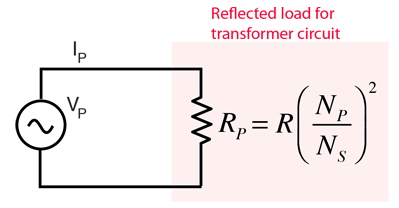# Reflected Load in a Transformer

When a voltage source supplies energy to a load through a transformer, the load as seen from the voltage source is different from the actual load because of the presence of the transformer. Considering a resistive load R on the secondary, the effective resistance as seen by the primary source will depend upon the turns ratio of the primary and secondary coils of the transformer. This effective resistance as seen by the voltage source is often called the "reflected load", evoking the image that the load resistor is "reflected" back across the transformer to be seen by the source. Once this reflected load is evaluated, the circuit can be considered like a simple resistive series circuit. The treatment here will assume an ideal transformer.Treating the circuit as if the primary were a simple series resistor RP, the effective value of that resistor can be calculated from Ohm's law and the transformer relationship.This "reflected load" approach provides the advantage of treating the circuit as a simple resistor circuit for the calculation of different load conditions.
 Transformer Calculation
Index

Transformer concepts

Magnetic field concepts

 HyperPhysics*****Electricity and magnetism R Nave
Go Back